Technical Article

# Understanding Electromagnetism

August 25, 2021 by Alex Roderick

## This article will examine the magnetic field produced by current-carrying straight conductors as well as that produced by coils.

Electromagnetism is a branch of physics that studies the relationship between electric currents and magnetic fields. When there is no current flow in a conductor, electrons move in a random manner. When a voltage is supplied to a conductor, creating a current flow, electrons will have a net movement in the opposite direction of the applied electric field. This causes their magnetic fields to align with each other. The magnetic fields combine and extend outside the conductor. Figure 1 shows the magnetic field lines at a distance of d from a straight wire carrying a current of I.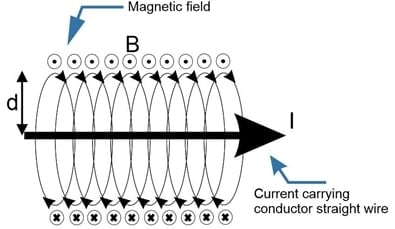## Magnetic Field Strength and Distance

In theory, the magnetic field around the conductor's circumference extends infinitely into space. If a compass is placed near the current-carrying conductor, the compass will indicate the polarity of the field. However, the greater the distance between the compass and the conductor, the weaker the field is on the compass. At some point, there is no effect, and the compass will align with the magnetic field of the earth.

A magnetic field can be detected at a larger distance if the current flowing through the conductor is increased. This shows that the magnitude of the magnetic field depends on both the current value and the distance from the conductor.

The magnetic field stretches the entire length of the conductor with concentric rings of magnetic force.

## Conductors

A conductor is a material with a low electrical resistance that allows electrons to easily pass through it. When current flows in opposite directions in two parallel current-carrying conductors, magnetic lines of force generated by the two currents are in the same direction between the conductors. This results in a force that tends to push the two conductors apart. If the current flow is reversed in one of the conductors, the direction of the magnetic lines of force surrounding the same conductor also reverses. In this case, the magnetic lines of force of the two currents are in the opposite direction and the two conductors attract each other(see Figure 2).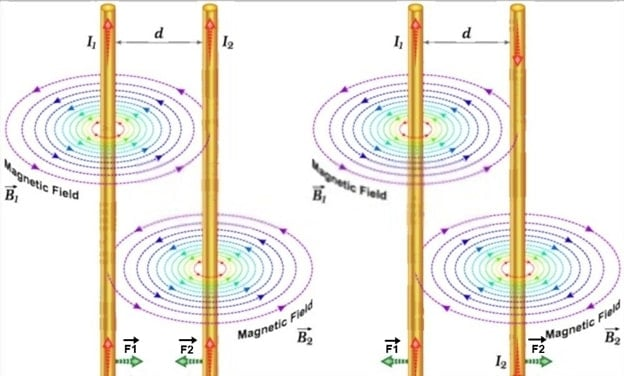## Right-Hand Rule

The right-hand rule can be used to determine the direction of magnetic lines of force around a current-carrying conductor. According to this rule, when a conductor is wrapped in the right hand with the thumb pointing in the direction of the current flow, the fingers indicate the direction of the magnetic lines of force. (see Figure 3).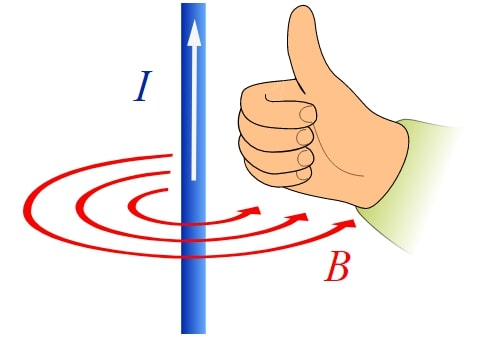##### Figure 3. The right-hand rule for determining the direction of the magnetic field produced by a current-carrying conductor. Image courtesy of Khanacademy.

When a straight, current-carrying conductor produces a magnetic field, the magnetic lines of force are concentric around the conductor, without detectable magnetic poles. This is indicated by moving a compass from below the conductor to above the conductor and observing the rotation of the compass needle. As the compass is moved in a circular motion at a given distance from the conductor, the needle swings to align with the magnetic lines of force perpendicular to the conductor.

An electromagnet with a north and south pole is created by forming a loop in a current-carrying conductor. The electromagnet's south pole attracts the compass's north pole (see Figure 4).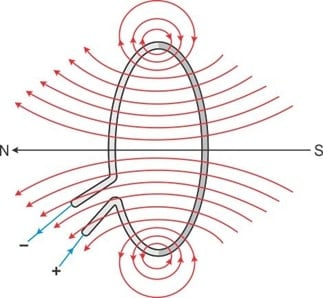## Coils

A coil is a circular wound wire made up of insulated conductors arranged to create magnetic flux lines. A coil offers considerable opposition to the passage of AC but very little opposition to DC. Coils may be wound loosely or tightly—the tighter the turns of a coil, the stronger the magnetic field surrounding the coil (see Figure 5).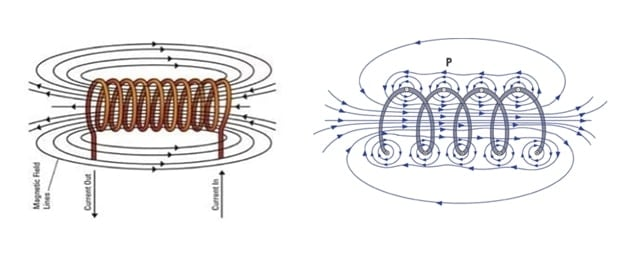##### Figure 5. Coils may be wound loosely or tightly. When the turns are tighter, the produced magnetic field becomes stronger. Image Courtesy of Maker Pro

Because there is current flow in the same direction in all of the turns of a coil, the magnetic field produced is similar to the magnetic field produced by parallel conductors with current flow in the same direction. Magnetic lines of force created between adjacent turns of the coil oppose each other and hence, the magnetic field between the turns is weakened. By winding the coil turns tightly, the magnetic field is made stronger, with more magnetic lines of force encircling the entire coil. Under this condition, the individual concentric magnetic force lines around the conductor join to encircle the entire coil.

When current flow is present through the coil, the coil has a magnetic field comparable to a permanent magnet. The right-hand rule for coils is used to determine the north and south polarities of the magnetized coil. To apply the right-hand rule to coils, grasp the coil with the right hand such that the fingers encircle the coil in the direction the electric current flows through the coil windings. Now, extend your thumb at a right angle to the fingers—the thumb will point toward the coil's north pole. If the direction of current is reversed, the polarity of the magnetic field also reverses. The right-hand rule is illustrated in Figure 6.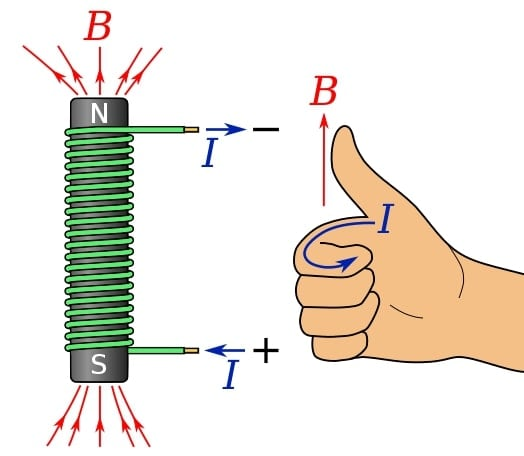##### Figure 6. The right-hand rule for determining the polarity of a coil. Image courtesy of Wikimedia.

Several factors affect the strength of a coil's magnetic field, including the number of conductor turns, amount of current flow through the coil, the ratio of coil's length to its width, and type of coil core material.

## Electromagnets

Electromagnets are coils with soft iron as their core material. The core material improves the magnetic field strength because soft iron offers a better path for magnetic lines of force than air and concentrates them into a smaller region. Once current is removed from the coil, the electromagnet loses its magnetic characteristics because soft iron has very little residual magnetism. The right-hand rule for coils is used to determine the polarity of an electromagnet's magnetic field.